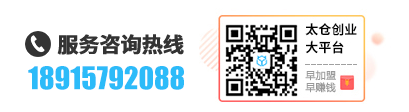# 太仓DedeCMS首页和列表页调用图片集多张图片dede:arclist 标签调用和dede:list 列表调用

[field:id function="Getimg(@me,80,80,7)" /]

{dede:field.id function="Getimg(@me,80,80,7)" /}

80和80和7分别是要显示图片的宽度(省略为110)和高度(省略为110)和调用张数（省略为所有((0))，

function Getimg(\$aid,\$imgwith=110,\$imgheight=110,\$num=0)

global \$dsql;

\$imgurls = '';

\$row =\$dsql->getone( "Select imgurls From `dede_addonimages` where aid='\$aid' ");

\$imgurls= \$row['imgurls'];

preg_match_all("/{dede:img (.*)} (.*){\/dede:img/isU",\$imgurls,\$wordcount);

\$count=\$count(\$wordcount);

if (\$num>\$count || \$num==0){\$num=\$count;}

for(\$i=0;\$i<\$num;\$i++){

\$imglist.="<li><img src=".trim(\$wordcount[\$i])." width=".\$imgwith." height=".\$imgheight."></li>";

return \$imglist;

}

•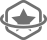品质保证
16年以上财税经验积累
获得国家中小企业投资

•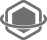专业实力
资深财税团队
专业会计团队

•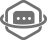安全无忧
2048位安全证书
银行级别的系统安全

•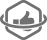多元服务
社保托管、税务代办
财务规划和咨询等增值服务

APP扫码下载